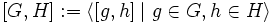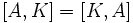# Commutator of a group and a subgroup implies normal

Jump to: navigation, search
This article describes a computation relating the result of the commutator operator on two known subgroup properties or properties of subsets of groups: (i.e., improper subgroup and subgroup), to another known subgroup property (i.e., normal subgroup)
View a complete list of commutator computations
This article gives the statement and possibly, proof, of an implication relation between two subgroup properties. That is, it states that every subgroup satisfying the first subgroup property (i.e., subgroup realizable as the commutator of the whole group and a subgroup) must also satisfy the second subgroup property (i.e., normal subgroup)
View all subgroup property implications | View all subgroup property non-implications
Get more facts about subgroup realizable as the commutator of the whole group and a subgroup|Get more facts about normal subgroup

## Statement

### Statement with symbols

Suppose$G$ is a group and$H$ is any subgroup. Then, the commutator$[G,H]$, defined as:$[G,H] := \langle [g,h] \mid g \in G, h \in H \rangle$

is a normal subgroup of$G$.

## Facts used

1. Subgroup normalizes its commutator with any subset: If$K \le G$ and$A$ is a subset of$G$, then$K$ normalizes the commutator$[A,K] = [K,A]$.

## Proof

Given: A group$G$, a subgroup$H$.

To prove: The subgroup$[G,H]$ is normal in$G$.

Proof: Apply fact (1) to$K = G$ and$A = H$. We get that$G$ normalizes$[G,H]$. Hence,$[G,H]$ is normal in$G$.